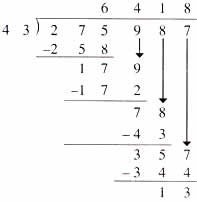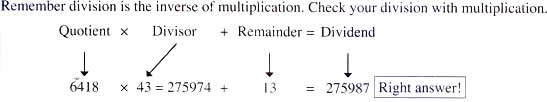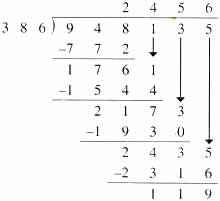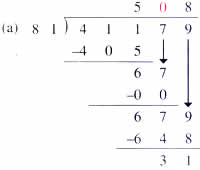Sunday , July 12 2020# NCERT 5th Class (CBSE) Mathematics: The Four Operations And Their Application

## DIVISION

### Properties of Division:

1. Any number divided by itself is 1.
2. Any number divided by 1 is the number itself.
3. The divisor can never be zero.

### Dividing by a 2-Digit Number### Dividing by a 3-Digit Number### Check with multiplication:

Quotient × Divisor + Remainder = Dividend

2456 × 368 = 948016 + 119 = 948135

### Zeros in the quotient## 10th Class CBSE Mathematics 2018-19

10th Class CBSE Mathematics 2018-19 Time: 3 hours M.M.: 80 Class: 10th Subject: Mathematics Date: 07/03/2019 …